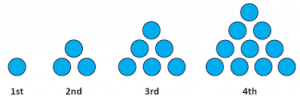# Sequences and Series: Introduction

A sequence is an ordered set of elements – usually numbers – and is generally written with large curly brackets:

{1st number, 2nd number, 3rd number …}

For example:

{10, 9, 8, 7, 6, …}

A sequence may be finite, in which case there are a set number of terms to the sequence. The following sequence starts at 10, ends at zero and has 11 terms. It is common to show the first three and the last two terms of a sequence – or as many terms are needed to demonstrate the pattern. This sequence generally demands a loud, booming voice and a sense of dramatic expectation:

{10, 9, 8, ….,1, 0}

A sequence that continues forever is an infinite sequence. The following sequence is a famous sequence known as the Fibonacci Sequence, named after the medieval Italian mathematician, Leonardo of Pisa. A simple internet search on the Fibonacci numbers will lead to several different and fascinating topics.

{0, 1, 1, 2, 3, 5, 8, …}

## Example 1

Sequences of shapes yield interesting numerical patterns. One such sequence is the triangle numbers, which can be created using marbles or gravel or anything you have to hand.Now, you can make the second term just by adding two stones to the first term, in which case you only need three stones to illustrate the first two terms. If you make the first term, then the second term separately, you will need 4 stones.

1. How many stones do you need to make the 10th term, all on its own?
2. How many stones do you need to make the first 10 terms separately?

Did you use any shortcuts or tricks to find the answers to these two questions?

## Example 2

Another famous sequence of shapes is the Koch Snowflake. The first shape is simply an equilateral triangle. The first three shapes are illustrated in the following applet.

1. We begin with an equilateral triangle. After trisecting the edges, we add three triangles (Snowflake 2). After trisecting the new edges, we add twelve triangles (Snowflake 3). If we were to trisect the edges one more time, how many triangles would we add?
2. Suppose the first triangle has side length 9 cm. The perimeter of the first iteration is 27 cm. Then, one equilateral triangle of side length 3 cm is drawn on each side. The perimeter of the second iteration is 36 cm (Snowflake 2). Next, twelve equilateral triangles with side length 1 cm are drawn on each edge. What is the perimeter of the third iteration?

On each iteration of the Koch Curve, we get a new value for the number of triangles, the perimeter of the snowflake and for the area of the snowflake.

Understanding the mathematics of basic sequences helps to analyze the sequences that are generated by the Koch Curve.

And once the snowflake has been analysed, mathematics presents us with a concept that at first sight seems to be quite absurd!